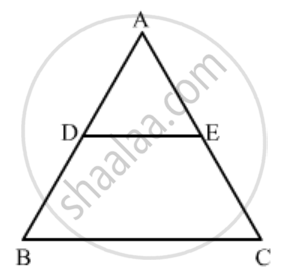Advertisement Remove all ads

# In ∆Abc, D and E Are Points on Side Ab and Ac Respectively Such that De || Bc and Ad : Db = 3 : 1. If Ea = 3.3 Cm, Then Ac = (A) 1.1 Cm (B) 4 Cm (C) 4.4 Cm (D) 5.5 Cm - Mathematics

MCQ

In ∆ABC, D and E are points on side AB and AC respectively such that DE || BC and AD : DB = 3 : 1. If EA = 3.3 cm, then AC =

#### Options

• 1.1 cm

• 4 cm

• 4.4 cm

• 5.5 cm

Advertisement Remove all ads

#### Solution

Given: In ΔABC, D and E are points on the side AB and AC respectively such that DE || BC and AD : DB = 3 : 1. Also, EA = 3.3cm.

To find: ACIn ∆ABC, DE || BC.

Using corollory of basic proportionality theorem, we have

(AD)/(AB)=(EA)/(AC)

(AD)/(AD+1/3AD)=3.3/(AC)

EC =4.4 cm

Hence the correct answer is C

Concept: Triangles Examples and Solutions
Is there an error in this question or solution?
Advertisement Remove all ads

#### APPEARS IN

RD Sharma Class 10 Maths
Chapter 7 Triangles
Q 9 | Page 132
Advertisement Remove all ads

#### Video TutorialsVIEW ALL 

Advertisement Remove all ads
Share
Notifications

View all notifications

Forgot password?
Course